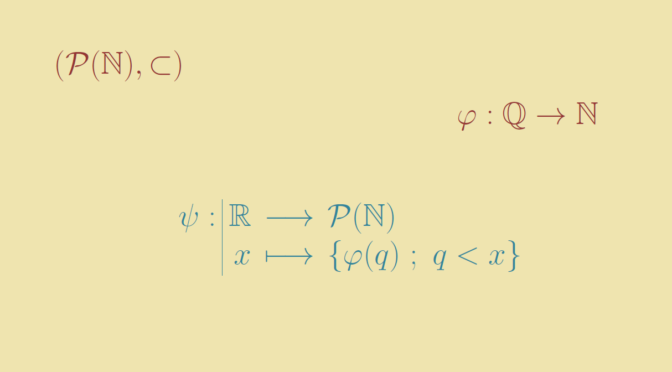# An uncountable chain of subsets of the natural numbers

Consider the set $$\mathcal P(\mathbb N)$$ of the subsets of the natural integers $$\mathbb N$$. $$\mathcal P(\mathbb N)$$ is endowed with the strict order $$\subset$$. Let’s have a look to the chains of $$(\mathcal P(\mathbb N),\subset)$$, i.e. to the totally ordered subsets $$S \subset \mathcal P(\mathbb N)$$.

### Some finite chains

It is easy to produce some finite chains like $$\{\{1\}, \{1,2\},\{1,2,3\}\}$$ or one with a length of size $$n$$ where $$n$$ is any natural number like $\{\{1\}, \{1,2\}, \dots, \{1,2, \dots, n\}\}$ or $\{\{1\}, \{1,2^2\}, \dots, \{1,2^2, \dots, n^2\}\}$

### Some infinite countable chains

It’s not much complicated to produce some countable infinite chains like $\{\{1 \},\{1,2 \},\{1,2,3\},…,\mathbb{N}\}$ or $\{\{5 \},\{5,6 \},\{5,6,7\},…,\mathbb N \setminus \{1,2,3,4\} \}$

Let’s go further and define a one-to-one map from the real interval $$[0,1)$$ into the set of countable chains of $$(\mathcal P(\mathbb N),\subset)$$. For $$x \in [0,1)$$ let $$\displaystyle x = \sum_{i=1}^\infty x_i 2^{-i}$$ be its binary representation. For $$n \in \mathbb N$$ we define $$S_n(x) = \{k \in \mathbb N \ ; \ k \le n \text{ and } x_k = 1\}$$. It is easy to verify that $$\left(S_n(x))_{n \in \mathbb N}\right)$$ is a countable chain of $$(\mathcal P(\mathbb N),\subset)$$ and that $$\left(S_n(x))\right) \neq \left(S_n(x^\prime))\right)$$ for $$x \neq x^\prime$$.

What about defining an uncountable chain?

### A chain having the cardinality of the continuum

Let’s first recall that the set of the rational numbers $$\mathbb Q$$ is countable. Hence one can find a bijection $$\varphi : \mathbb Q \to \mathbb N$$ (see following article).

Now consider the set of real numbers $$\mathbb R$$ which has the cardinality of the continuum $$\mathfrak c = 2^{\aleph_0}$$. For any real $$x \in \mathbb R$$, we can define the set of rationals $$\psi(x) = \{q \in \mathbb Q \ ; \ q \lt x\}$$. Then $$\varphi [\psi(x)]$$ is a subset of $$\mathbb N$$.

$$\left(\varphi [\psi(x)]\right)_{x \in \mathbb R}$$ is a chain as for $$x \lt y$$ we have $$\psi(x) \subset \psi(y)$$. It has the cardinality of the continuum as $$\psi$$ is one-to-one.